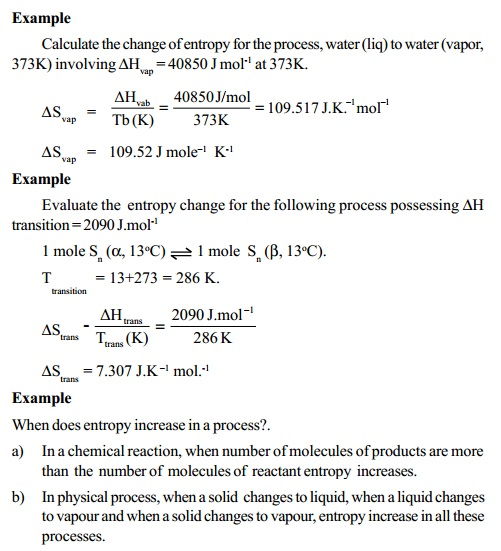Home | | Chemistry | Characteristics of entropy 'S'

# Characteristics of entropy 'S'

The term 'S' entropy is evolved from the formulation of II law of thermodynamics as a thermodynamic state function.

Characteristics of entropy 'S'

i.                        The term 'S' entropy is evolved from the formulation of II law of thermodynamics as a thermodynamic state function.

ii.                        Entropy change 'DS' of a system under a process is defined as the constant equal to the ratio of the heat change accompanying a process at constant temperature to the temperature of the system under process. The process should be reversible at that temperature.

DSrev = Dq rev / T(K)

Heat, q is not a state function , But for a reversible process Dq = (q2-q1)  divided by temperature (T) of the process is a state function.

iii.                        A spontaneous process is accompanied by increase in the 'disorder' (or) 'randomness' of the molecules constituting the system. Entropy increases in all spontaneous processes. Hence entropy may be regarded as a measure of disorder (or) randomness of the molecules of the system.

iv.                        When a system undergoes a physical (or) a chemical process, there occurs a change in the entropy of the system and also in its surroundings. This total change in the entropy of the system and its surroundings is termed as the entropy change of the universe brought about by the process. For an isothermal process (T=constant), the entropy change of the universe during a reversible process is Zero.

The entropy of the universe increases in an irreversible process.

v.            The energy of the universe remains constant although the entropy of the universe tends to a maximum.

vi.            For a spontaneous process, at constant T, DS is positive (DS > 0).

DS is positive (DS > 0). For an equilibrium process, DS is zero.

For a non spontaneous process,

DS is negative or (DS < 0).

vii) Units of entropy: The dimension of entropy are energy in terms of heat X temperature-1. The entropy is expressed as calories per degree which is referred to as the entropy units (eu). Since entropy also depends on the quantity of the substance, unit of entropy is calories per degree per mole (or) eu. per mole. cgs units of entropy is cal.K-1 denoted as eu. The SI unit is JK-1 and denoted EU. 1 eu = 4.184 EU.

viii)     Entropy change is related to enthalpy change as follows:

For a reversible and isothermal process,

DSrev = Dq rev / T

Since DH is the heat absorbed (or) evolved in the process at constant T and pressure P.DS is also calculated from DH as DS = DH / T where T is the temperature of the process involving DH, amount of enthalpy change, at constant pressure.Standard Entropy

The absolute entropy of a pure substance at 25 o C (298 K) and 1 atm pressure is called the standard entropy, S o . The standard entropies of all substances either elements or compounds at any temperature above 0 o K always have positive values.

When the standard entropies, S o of various substances are known, the standard entropy change of a chemical process or reaction is written as

DS o = S S o products - S S o reactants

This DS o is the standard entropy change of the reaction.

Standard entropy change of formation, DS o f is defined as the entropy of  formation of 1 mole of compound from the elements present in the standard conditions. DS o fcan be calculated for chemical compounds using the S o values of elements from which the compound is formed. DS o f    compound   =  SS o compound - S S o elementsStudy Material, Lecturing Notes, Assignment, Reference, Wiki description explanation, brief detail
11th 12th std standard Class Organic Inorganic Physical Chemistry Higher secondary school College Notes : Characteristics of entropy 'S' |